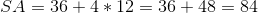## Example Questions

### Example Question #5 : How To Find The Surface Area Of A Pyramid

What is the surface area of a square pyramid with a height of 12 in and a base side length of 10 in?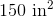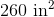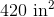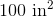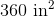Explanation:

The surface area of a square pyramid can be broken into the area of the square base and the areas of the four triangluar sides. The area of a square is given by: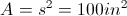The area of a triangle is: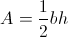The given height of 12 in is from the vertex to the center of the base. We need to calculate the slant height of the triangular face by using the Pythagorean Theorem: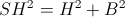where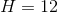and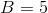(half the base side) resulting in a slant height of 13 in.

So, the area of the triangle is: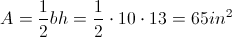There are four triangular sides totaling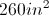for the sides.

The total surface area is thus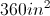, including all four sides and the base.

### Example Question #3 : Pyramids

Consider a square-base pyramid,. If one of the triangular faces ofis analyzed individually and it is found to have a base ofand a height of. What is the total surface area of?Explanation:

The surface area of this pyramid is equal to the area of the square base plus the areas of each of the four triangular sides.

We are given the base and height of the triangular faces.

We can use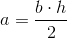and plug in 3 and 6, giving us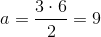.

Because the base of the triangular faces is equal to 3, we know the area of the square base is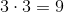.

Therefore, our total surface area is equal to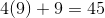### Example Question #4 : Pyramids

The largest of the Great Egyptian Pyramids has a base length and width of 230 metrers and a height of 147 meters. What is the surface area of this rectangular pyramid in scientific notation?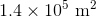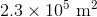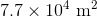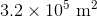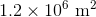Explanation:

The equation for surface area of a rectangular pyramid is as follows: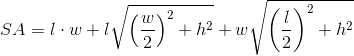Plug in the values for length, width and height: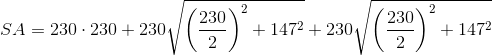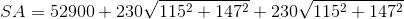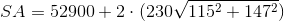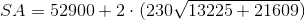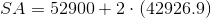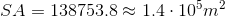### Example Question #5 : Pyramids

Find the surface of a isoceles pyramid whose height is, base side length is, and slant height is.Explanation:

To find surface area of a pyramid, simply find the surface area of each of the faces and add them together.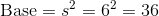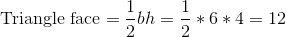Since there are 4 trangular faces, we have to multiply that surface area by 4. Thus,